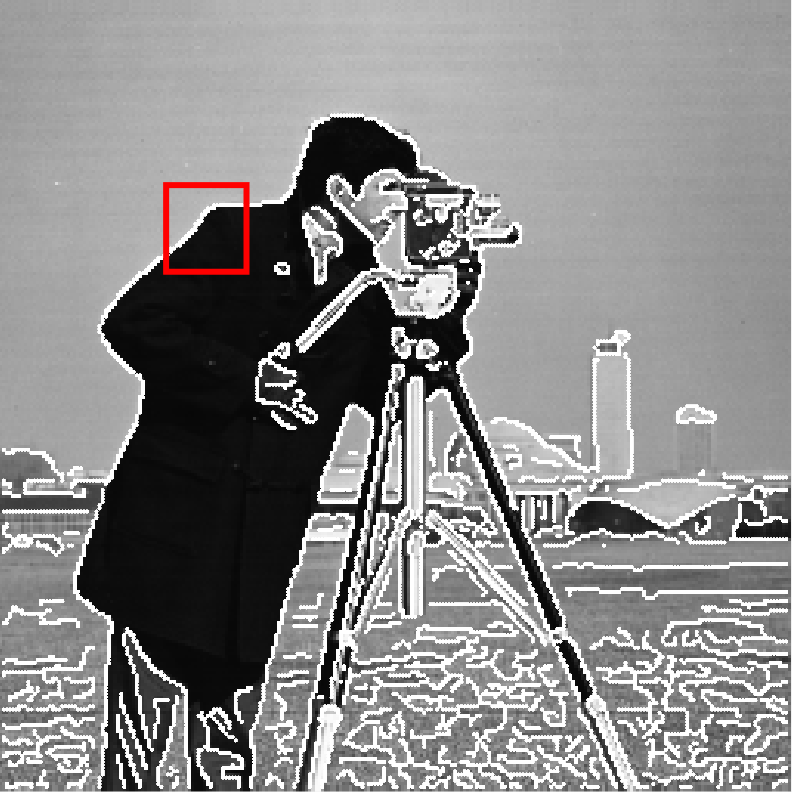# Save an image with a plot

조회 수: 2(최근 30일)
Alejandro Fernández 2020년 4월 9일
댓글: Ameer Hamza 2020년 4월 9일
Hey, see if anyone can fix a problem I have. I add an example using the sample image 'cameraman.tif', the image of the real code is another one but to get an idea it works perfectly.
I need to highlight the detected outline, so what I came up with was to get the pixel coordinates and represent them on top of the image by saying that these points are thicker.
Also, I make a crop of the image and I need to show in the original image the crop rectangle used.
When I save it, I want the highlighted pixels to look white and the square to look any other color, for example red.
Do you have any ideas on how to make the image as tight as possible so that the white pixels look white and not black?
Translated with www.DeepL.com/Translator (free version)
BW = edge(I,'canny');
[J,rect2] = imcrop(BW);
[r,c] = find ( BW == 1 );
pP = [c r];
f = figure;
imshow(I)
hold on
plot(pP(:,1),pP(:,2),'.w','MarkerSize',5)
rectangle('Position',rect2,'EdgeColor','red','LineWidth',3)
print('TH.tif','-dtiff')
What i want to obtainWhat i obtain using print.

댓글을 달려면 로그인하십시오.

### 채택된 답변

Ameer Hamza 2020년 4월 9일
Try this
BW = edge(I,'canny');
[J,rect2] = imcrop(BW);
[r,c] = find ( BW == 1 );
pP = [c r];
f = figure;
ax = axes;
imshow(I)
hold on
plot(pP(:,1),pP(:,2),'.w','MarkerSize',5)
rectangle('Position',rect2,'EdgeColor','red','LineWidth',3)
frame = getframe(gca);
imwrite(frame.cdata, 'TH.tif')
If you are using R2020a, then MATLAB also introduced exportgraphics
BW = edge(I,'canny');
[J,rect2] = imcrop(BW);
[r,c] = find ( BW == 1 );
pP = [c r];
f = figure;
ax = axes;
imshow(I)
hold on
plot(pP(:,1),pP(:,2),'.w','MarkerSize',5)
rectangle('Position',rect2,'EdgeColor','red','LineWidth',3)
exportgraphics(gca,'TH.tif')
##### 댓글 수: 2표시숨기기 이전 댓글 수: 1
Ameer Hamza 2020년 4월 9일

댓글을 달려면 로그인하십시오.

R2020a

### Community Treasure Hunt

Find the treasures in MATLAB Central and discover how the community can help you!

Start Hunting!